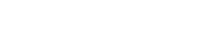The Pattern in prime numbers

# 06 Feb The Pattern in prime numbers

If you have taken some math tuition on number theory, you should be familiar with the concept of prime numbers. Prime numbers are those numbers which are only divisible by 1 and that number itself. Some examples of prime numbers are 2, 3,7,11 13 etc. These numbers can only be divided by themselves or 1 without producing a remainder.

Prime numbers are one of the most beautiful things in mathematics. They are also quite mysterious in some sense. For thousands of years, many great minds have worked with them to uncover some of their mysteries. The first and foremost thing that the mathematicians wanted to find about prime numbers is a definitive pattern in their distribution. For example, let us consider the following sequences of numbers:

Sequence 1: 2, 4, 6, 8, 10…..
Sequence 2: 2, 4, 8, 16….
Sequence 3: 1, 1,2,3,5,8,13…

In all of the above 3 sequence of numbers, we can see a definitive pattern. In the first sequence, all numbers are separated by an interval of 2 i.e. we get the next number by adding 2 to the current number. All the numbers generated in this sequence will be even if we follow the same pattern, given we start with an even number. If we take the example of the second sequence, each number (apart from the first number of course) is double of the previous number i.e we generate the next number by multiplying 2 to the current number. The third sequence is known as the Fibonacci sequence and there is a definitive pattern to it as well. Can you see the pattern? Well, each number, apart from the first two numbers (i.e. 1 and 1), is the sum of the previous 2 numbers. For example, 3 is 2+1 and 13 is 8+5. As we can clearly see, there is a definite pattern. The mathematicians wanted to find the same thing for prime numbers; they wanted a pattern using which prime numbers could be generated and predicted. Although there was no lack of trying and many great minds of all time wrestled with this particular problem, till date, we have failed to find such a definitive pattern.

Leonhard Euler, one of the greatest mathematicians of all time, in fact came up with a formula for generating prime numbers. Those of you who have taken some math tuition on algebra or number theory might already be familiar with this formula. The formula goes as follows:If we plug-in the values of n, we will indeed generate primes – well, at least in some cases. For example, when we plug-in n=1, we get 41 – prime. If we plug-in n=2, we get 43 – again a prime. If we plug-in n=3, we get 47, a prime!! In fact, this formula works all the way up to 40. The point it fails at is when n=41 when the value of the expression becomes 1763 which is not prime because this number is the product of 43 and 47 and hence devisable by both of them. As a result, although the formula shows some promise early on, it fails at a point. In fact, this formula potentially generates an infinitely many composite numbers. Although we have failed to find a general pattern which predicts the distribution of prime numbers, it is important to mention here that we have been able to find certain patterns which hold true in certain cases. For example, the Ulam spiral, the prime number chains, Gilbreath’s conjecture and many others are examples where such patterns are observed.

Number pattern is a familiar topic for maths tuition students in Singapore. Though it starts from the basic primary maths tuition classes in Singapore, maths tuition students are well aware of number patterns. One of the very popular maths questions many years ago was to find the sum of numbers from 1 to 100. This question was for 12 year olds students taking the maths examination.

Automated page speed optimizations for fast site performance